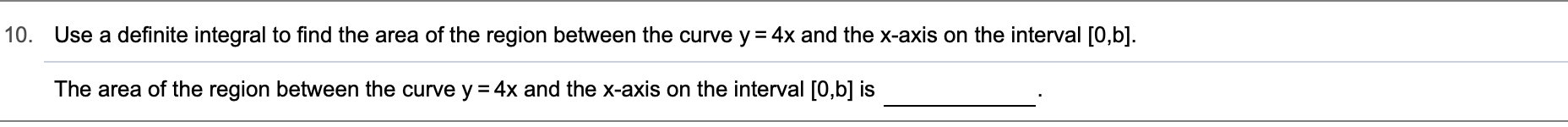# 10.Use a definite integral to find the area of the region between the curve y 4x and the x-axis on the interval [0,b].The area of the region between the curve y 4x and the x-axis on the interval [0,b] is

Question
22 views

Can you help with this problem step by step?help_outlineImage Transcriptionclose10. Use a definite integral to find the area of the region between the curve y 4x and the x-axis on the interval [0,b]. The area of the region between the curve y 4x and the x-axis on the interval [0,b] is fullscreen
check_circle

Step 1

We know that area of the region bounded by curve y=f(x) and x-axis from x=a to x=b is

Step 2

Thus , area of region between y=4x ,...

### Want to see the full answer?

See Solution

#### Want to see this answer and more?

Solutions are written by subject experts who are available 24/7. Questions are typically answered within 1 hour.*

See Solution
*Response times may vary by subject and question.
Tagged in
MathCalculus

### Integration